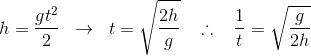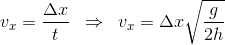# How to derive this equation of missile launch?

CarlosK
Hi All,
Question: How to derive these equations of missile launch?

v=∆x√(g/2H)

v=√(2gh/β+1)

I need to use these formulas:
∆y=voyt - (gt²)/2
∆x=vxt
K=(mv²)/2 + (Iω²)/2
∆UG=mg∆y
I=βmr²
β=2/5

Thanks, Carlos.

Last edited:

Mentor
They seem to be derived for a specific problem statement. How exactly are they used?

CarlosK
The exercise asked to deduce these formulas.

Mentor
Without any other context or diagram? Then the problem statement is really bad.

Homework Helper
Dearly Missed
Hi All,
Question: How to derive these equations of missile launch?

v=∆x√(g/2H)

v=√(2gh/β+1)

I need to use these formulas:
∆y=voyt - (gt²)/2
∆x=vxt
K=(mv²)/2 + (Iω²)/2
∆UG=mg∆y
I=βmr²
β=2/5

Thanks, Carlos.

When parsed according to standard rules for reading mathematical expressions, the second formula above means
$$v = \sqrt{ \frac{2gh}{\beta} + 1}$$
Is that really what you wanted, or did you mean
$$v = \sqrt{ \frac{2gh}{\beta + 1}} ?$$
If the latter, you need to use parentheses, like this: v = √ (2gh/(β+1)).

CarlosK
Sorry..
v = √ (2gh/(β+1)) is correct. Thanks

CarlosK
I got this v = √ (2gh/(β+1)).
I need this v=∆x√(g/(2H))

CarlosKby: Euclides (http://pir2.forumeiros.com/)

Mentor
Combining those equations only makes sense if ##\Delta x=h## (they have to apply to the same time t), and if vx is the average velocity during ascent (or descent).